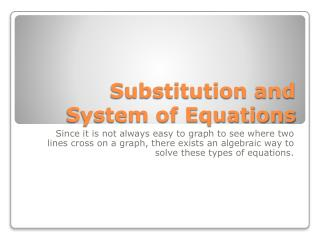DownloadDownload PresentationSubstitution and System of Equations

# Substitution and System of Equations

Télécharger la présentation## Substitution and System of Equations

- - - - - - - - - - - - - - - - - - - - - - - - - - - E N D - - - - - - - - - - - - - - - - - - - - - - - - - - -
##### Presentation Transcript

1. Substitution and System of Equations Since it is not always easy to graph to see where two lines cross on a graph, there exists an algebraic way to solve these types of equations.

2. A = 3 B=-2 C=1/2 AB B÷C + A

3. If the lines cross, they will intersect in one common point. Sometimes the intersecting point is not exact. You might have fraction answers. Solving equations using substitution in an algebraic way will enable you to find any answer no matter how weird. You will algebraically solve for one of the letters and then substitute back in to get the other letter value. The two letter values are the point of intersection where the two graph lines would cross. You will solve these questions using the algebraic method. You can verify your result using the calculator if you wish. Don’t forget it is still possible to have to have no solution or many solutions. The final answer will be either a coordinate point (x,y), no solution, or many solutions.

4. The key to solving using substitution is to have one of the variables(letters) equal to the rest of the equation. y = -6 4x + y = 2

5. y = 2x 4x – y = -4

6. y= 2x + 6 y= -x - 3

7. Sometimes you will need to rearrange to get a letter equal to the rest of the equation. 2x + y = -4 5x + 3y = -6

8. You can find more examples in the algebra online materials. Go to www.glencoe.com to the extra examples or the personal tutor in the algebra book 2008. Look at chapter 5 lesson 2.

9. Harder Substitution with Systems of Equations Examples

10. y = 2 – x 2y – x = 10

11. -.2x + y = .5 .4x + y = 1.1

12. Don’t forget about no solution or many. x + y = 4 x + y = 1

13. 3x + y = 3 2y = -6x + 6

14. Application Problem: Angles X and Y are supplementary. The measure of angle X is 24 degrees greater than the measure of angle Y. Find the measures of each angle.

15. You can find more examples in the algebra online materials. Go to www.glencoe.com to the extra examples or the personal tutor in the algebra book 2008. Look at chapter 5 lesson 2.